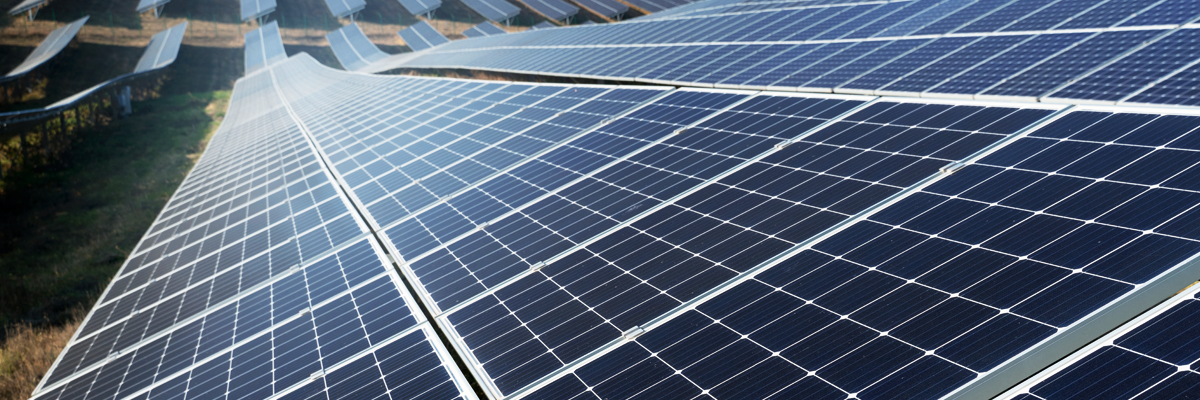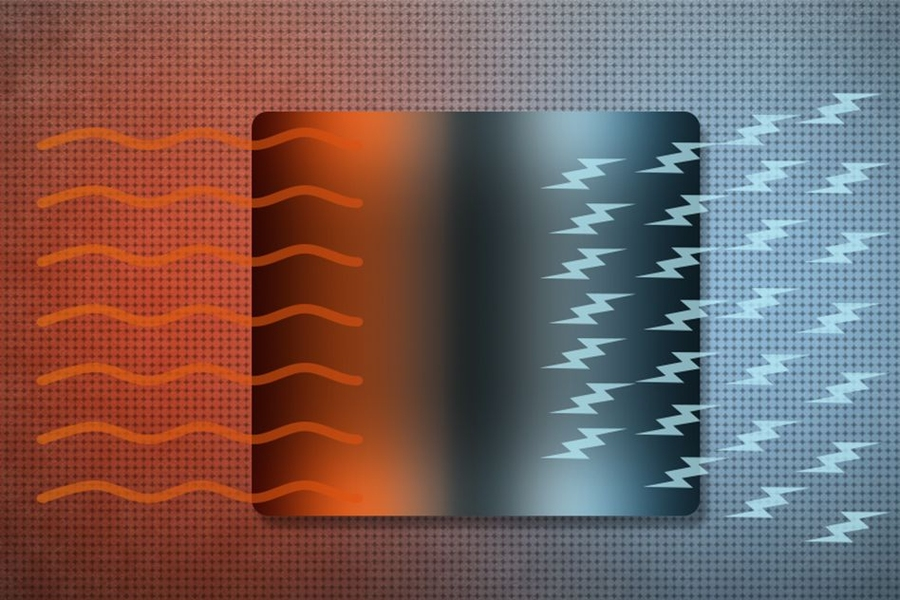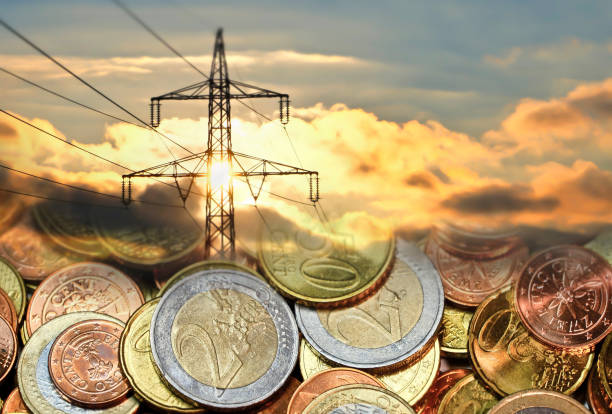Full 1

Dedicated to the promotion &
development of renewable energy.#### How much electricity is used to heat water?

The amount of electricity consumed in heating water is fundamental to understanding the overall financial equation.

The simple formula is to take the volume of water to be heated, the temperature of the water when cold and when hot, called the ‘temperature differential’, and the amount of electricity used to heat the volume by the temperature differential.

Volume ×Temperature Differential ÷ 860 = kWh (electricity used)

The cold water in Johannesburg is as low as 10 ºC in winter and as high as 22 ºC in mid-summer with an average of 16 ºC. For a hot water thermostat setting in the electric geyser of 60 ºC this is an average temperature differential of 44 ºC.For example:

100 Liters × 44 °C ÷ 860 kWh/(Litres K) = 5.11 kWh

If the price of electricity is R2.00 per kWh the cost of heating the tank of 100 litres from cold to hot is
4.5kWh × R2.00 = R9.00.